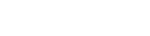# DC Circuit Analysis

For this, we will simplify our circuit further by shorting out inductors, and open-circuiting capacitors. So any loop containing a capacitor will no longer be a loop. And some loops will have inductors removed. We will only have resistor networks driven by voltage sources.

``````func get_dc_loops(loops_):
var regex = RegEx.new()
regex.compile("^L\\d*")
var dc_loops_ = []
for loop_ in loops_:
var is_loop = true
var dc_loop = []
for part in loop_:
if part.begins_with("ECap") or part.begins_with("C"):
is_loop = false
break
# skip inductors
if  not regex.search(part):
dc_loop.append(part)
if is_loop:
dc_loops_.append(dc_loop)
return dc_loops_
``````

Then we may apply each DC voltage source in turn to the circuit with the other voltage sources shorted.

Each loop should have no more than one voltage source that will be grounded at one end for the purposes of the analysis. Multiple series voltage sources could be combined into one, but then, various voltage offsets would need to be applied to the eventual solution.

Then we may traverse the loops, to create linear equations for the currents at each node (KCL) and for voltages (KVL).

We should be able to solve the equations using matrix calculations to get values for the currents and then assign current and voltage values to the circuit nodes.

And, we need to sum the currents flowing through common parts of any subsequent runs involving other voltage sources according to the Superposition Theorem. Sounds difficult, but we just add to the current value stored in a part already.

Finally, assign voltages and currents to the inductors and voltages to the capacitors of the original circuit. The voltages would be relative to the ground point of the circuit.

This solution could be revisited later to add features to handle non-linear devices such as diodes, and transistors.

The main difficulty that I see with this scheme is creating and operating on the matrices. Here is a potentially useful article on the topic: Solving 2x2, 3x3, 4x4 and 5x5 Systems of Linear Equations on a Computer.

Maybe the AC analysis will prove too hard for me to do? But let’s see.

## More Devlog entries

Most recent first